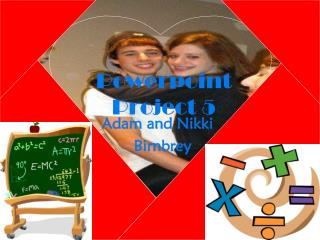DownloadDownload PresentationPowerpoint Project 5

# Powerpoint Project 5

Download Presentation## Powerpoint Project 5

- - - - - - - - - - - - - - - - - - - - - - - - - - - E N D - - - - - - - - - - - - - - - - - - - - - - - - - - -
##### Presentation Transcript

1. Powerpoint Project 5 Adam and Nikki Birnbrey

2. Properties of Equality to Know and Love • Addition Property- If a=b, then a+c= b+c • Subtraction Property- If a=b, then a-c=b-c • Multiplication Property- If a=b, then ac=bc • Division Property- If a=b and c doesn’t = 0 Then a/c= b/c • Substitution Property- If a=b you may replace a with b in any equation containing a in the result

3. Equivalence Properties of Equality to Know and Love • Reflexive Property- For any real number a, a=a • Symmetric Property- For all real numbers a and b, if a=b, then b=a • Transitive Property- For all real numbers a, b, and c, if a=b and b=c, then a=c

4. Equivalence Relation To Know And Love= any relation that satisfies these equivalence properties • Reflexive Property- Figure A=Figure A A • Symmetric Property- If Figure A=Figure B, then Figure B=Figure A A • Transitive Property- If Figure A=Figure B and Figure B=Figure C, Then Figure A=Figure C A B C

5. Overlapping Theorem to Know and Love • Overlapping Segments- Given a segment with points A, B, C, & D arranged as shown the following statements are true: 1. If AB=CD then AC=BD 2. If AC=BD then AB=CD • Overlapping Angles- Given Angle AED with points B and C in its interior as shown, the following statements are true: 1. If Angle AEB=Angle CED then ? 2. If Angle AEC=Angle BED then ? A B C D A B C D E

6. Vertical Angles Theorem to Know and Love • If two angles form a pair of vertical angles, then they are congruent. Given: angle 1 and 2 are vertical angles Prove: Angle 1 and 2 are congruent Statements Reasons 4 1 2 3 Given Linear pair property Substitution property of equality Subtraction property of equality Angle 1 and 2 are vertical angles 2. Angle 1 + angle 2= 180 Angle 2 + angle 3= 180 3. Angle 1+ angle 3= angle 2 + angle 3 4. Angle 1= angle 2 (1=2)

7. Congruence Supplements Theorem to Know and Love • If two angles are supplements of congruent angles then the two angles are congruent Given: Angle 1=Angle 3, Angle 1 and angle 2 are supplementary, Angle 3 and 4 are supplementary Prove: Angle 2=Angle 4 Statements Reasons 3 4 1 2 1. <1+<2=180 <3+<4=180 2. <1+<2=<3+<4 3. <1=<3 4. <1+<2=<1+<4 5. <2=<4 Definition of Supplementary Angles Transitive Given Substitution Property Subtraction Property

8. More Theorems to Know and Love • Theorem- Reflection across two parallel lines is equivalent to a translation of twice the distance between the lines and in a direction perpendicular to the lines • Theorem- Reflection across two intersecting lines is equivalent to a rotation about the point of intersection through twice the measure of the angle between the lines

9. Vocabulary to Know and Love • Equivalence relation= Any relation that satisfies the Reflexive, Symmetric, and Transitive Properties • Inductive reasoning= The process of forming conjectures that are based on observations • Paragraph proof= A form of a proof in which one’s reasoning is explained in paragraph form, as opposed to a two-column proof • Theorem= A statement that has been proved deductively • Two column proof= A proof in which the statements are written in the left-hand column and the reasons are given in the right-hand column • Vertical angles= The opposite angles formed by two intersecting lines

10. Practice to Know and Love(and a pooton of it) • Vertical Angles: Definition, illustrated examples, and an interactive practice quiz

11. A real pooton of Practice to Know and Love (yeah math) • Given: 15x-5=10x+15 (use properties to prove) • Prove the Overlapping Segments Theorem Given: WX=YZ; Prove WY=XZ W X Y Z segment additon postulate ______property WX=YZ WX+XY= YZ+XY WX=XY=WY

12. ….Extra poo • Identify the properties of equality that justify the conclusion. <B= <C; <C= <D <B= <D ________ AB=CD; CD=AB_______ AB+BC= BC+ CD; AC=BD_______

13. Finding Values: Problemas (To Know and Love) Find x. 3X- 60 120-6X X+40 10X